# Semi-linear mapping

(diff) ← Older revision | Latest revision (diff) | Newer revision → (diff)

A mappingfrom a (left) moduleinto a (left) moduleover the same ring, satisfying the conditions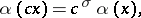where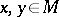,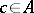andis some automorphism of. One says thatis semi-linear relative to the automorphism. A semi-linear mapping of vector spaces over the fieldrelative to complex conjugationis also known as an anti-linear mapping. A semi-linear mapping of an-moduleinto itself is known as a semi-linear transformation.

Example. A homothety of an-module, i.e. a mapping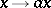(), whereis a fixed invertible element of, is a semi-linear mapping relative to the automorphism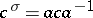.

Many properties of linear mappings and homomorphisms of modules remain valid for semi-linear mappings. In particular, the kernel and image of a semi-linear mapping are submodules; semi-linear mappings of free modules with finite bases are completely determined by their matrices; one can define the rank of a semi-linear mapping of vector spaces, which is equal to the rank of its matrix; etc.

How to Cite This Entry:
Semi-linear mapping. Encyclopedia of Mathematics. URL: http://encyclopediaofmath.org/index.php?title=Semi-linear_mapping&oldid=18709
This article was adapted from an original article by A.L. Onishchik (originator), which appeared in Encyclopedia of Mathematics - ISBN 1402006098. See original article